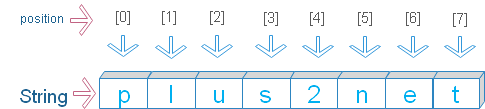# Reversing a string in Python

There are different ways to reverse a string.

## By using reversed()

We can reverse the sequence of all the elements of any iterable object by using using reversed() function . String method join() creates a string by joining all the elements.
``````str='plus2net'
str_rev=''.join(reversed(str))
print(str_rev)``````
Output
``ten2sulp``

## By looping & length

By using for loop we can reverse a sting. The function len() will return the length of the string. Using the length of the string we can start from the last position char and come upto the first char.
``````str='plus2net'
n=len(str) # length of the string
for i in range(n-1,-1,-1):
print(str[i],end='')``````
Output
``ten2sulp``

## By using string sliceThis is the shortest code to reverse a string. ( watch the last line only )
The first position of a string is 0 and last position is -1. The last line in below code will reverse the string as we are asking for full string starting from last (right most ) position.
``````str='plus2net'
print(str[:2]) # first two chars from left # pl
print(str[:-2]) # except last two chars # plus2n
print(str[-1]) # last position # t
print(str[::]) # full string starting from left # plus2net
print(str[::-1]) # from last position full string # ten2sulp``````

## for loop

All strings are iterable object so we can use for loop to iterate through the string.
``````str='plus2net'
x=''
for i in str:
x=i+x
print(x)``````
Output ( we are adding incremental char to left of the string x )
``ten2sulp``

Subscribe to our YouTube Channel here

## Subscribe

* indicates required
Subscribe to plus2netplus2net.com